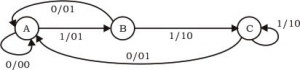# GATE | GATE-CS-2002 | Question 30

The Finite state machine described by the following state diagram with A as starting state, where an arc label is x / y and x stands for 1-bit input and y stands for 2- bit output(A) Outputs the sum of the present and the previous bits of the input.
(B) Outputs 01 whenever the input sequence contains 11.
(C) Outputs 00 whenever the input sequence contains 10.
(D) None of these

Explanation:
We assume the input string to be 1101.

1. (A, 1) –> (B, 01)
Here, previous input bit + present input bit = 0 + 1 = 01 = output

2. (B, 1) –> (C, 10)
Here, previous input bit + present input bit = 1 + 1 = 10 = output

3. (C, 0) –> (A, 01)
Here, previous input bit + present input bit = 1 + 0 = 01 = output

4. (A, 1) –> (B, 01)
Here, previous input bit + present input bit = 0 + 1 = 01 = output

Thus, option (A) is correct.

Please comment below if you find anything wrong in the above post.

Quiz of this Question

My Personal Notes arrow_drop_up
Article Tags :

Be the First to upvote.

Please write to us at contribute@geeksforgeeks.org to report any issue with the above content.